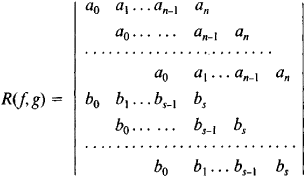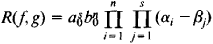# Resultant

Also found in: Dictionary, Thesaurus, Wikipedia.

## resultant

Maths Physics a single vector that is the vector sum of two or more other vectors
Collins Discovery Encyclopedia, 1st edition © HarperCollins Publishers 2005
The following article is from The Great Soviet Encyclopedia (1979). It might be outdated or ideologically biased.

## Resultant

an algebraic expression used to solve systems of algebraic equations. The resultant of the two polynomial equations f(x) = + • • • + a0xn + … + an = 0 and g(x) = b0xs + … + bs = 0 (a0 and b0 may vanish) is the determinantwhere the empty positions are occupied by zeros. The determinant has i rows containing the coefficients a0, a1, …,an and n rows containing the coefficients b0, b1,…. b3, If a0 ≠ 0 and b0 ≠ 0, thenwhere α1, α2, …, αn are the roots of the equation f(x) = 0 and β1, β2, …, βs are the roots of the equation g(x) = 0. The resultant vanishes if, and only if, the two equations have a common root or their leading coefficients are both equal to zero.

Let there be given the two equations P(x,y) = 0 and Q(x,y) = 0, where P and Q are polynomials in x and y. Suppose these polynomials are arranged according to powers of x, and the resultant of the polynomials obtained is equated to zero. An equation in y is then obtained of degree at most sn. Here, η is the degree of P in x and y, and s is the degree of Q. If x = x0, y = y0 is a solution of the given system of equations, then y = y0 is a root of the equation R(f, g) = 0. The process of solving a system of two equations is thereby reduced to the solution of a single equation.

The resultant of a polynomial equation and its derivative is equal, except possibly in sign, to the discriminant of the polynomial equation. If the discriminant is equal to zero, the polynomial equation has multiple roots.

### REFERENCE

Kurosh, A. G. Kurs vysshei algebry, 10th ed. Moscow, 1971.

## Resultant

The resultant of a system of forces is a force equivalent to the given system and equal to the vector sum of the forces: R = ΣFk. A system of forces applied at the same point always has a resultant if R ≠ 0. Any other system of forces applied to a body has a resultant, if R ≠ 0, when the moment of the system either is equal to zero or is perpendicular to R. In this case, the system of forces may be replaced by the resultant of the forces only when the body may be regarded as perfectly rigid. The system may not be replaced when, for example, internal forces are to be determined or when other problems that require the deformation of the body to be taken into account are to be solved. A couple and a system of two forces that are not coplanar are examples of systems of forces that do not have resultants.

## resultant

[ri′zəl·tənt]
(mathematics)
For a set of polynomial equations, a function of the coefficients which equals zero if the equations have at least one solution. Also known as eliminant.
McGraw-Hill Dictionary of Scientific & Technical Terms, 6E, Copyright © 2003 by The McGraw-Hill Companies, Inc.
References in periodicals archive ?
2012) can be used to creating a model of AE resultant signal that forms during CM wear.
It is easily seen that the resultant closed loop system is impulse free; that is, the impulse is eliminated by the derivative output feedback.
The TG-DTA curves of resultant 2 in air from room temperature to 1023 K are shown in Figure 1.
Des unites sont preparees pour se rendre immediatement sur les tas, en cas d'accidents resultant des taches d'huile.